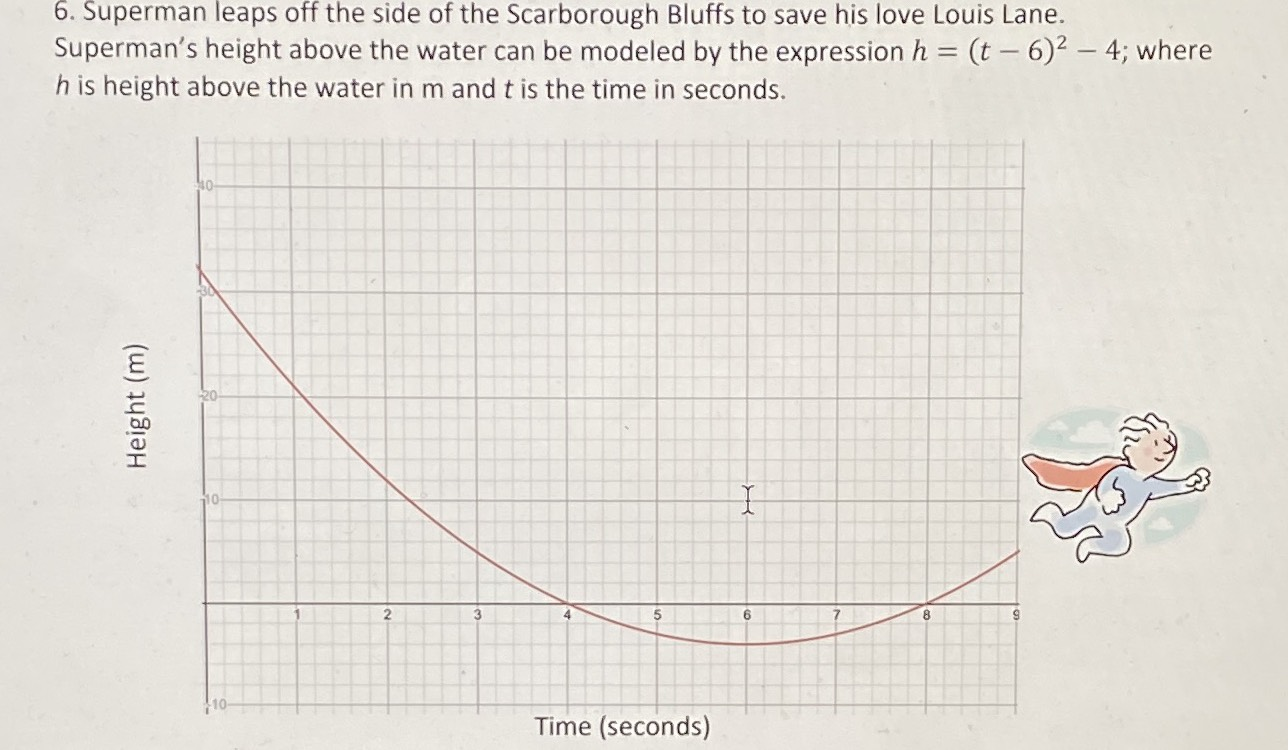### ¿Todavía tienes preguntas de matemáticas?

Pregunte a nuestros tutores expertos
Algebra
Pregunta6. Superman leaps off the side of the Scarborough Bluffs to save his love Louis Lane. Superman's height above the water can be modeled by the expression $$h = ( t - 6 ) ^ { 2 } - 4$$ ; where $$h$$ is height above the water in $$m$$ and $$t$$ is the time in seconds.